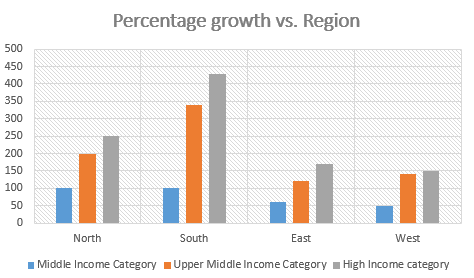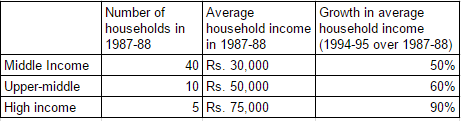# CAT 1998 Question Paper

Instructions

Answer the questions based on the following information. The following bar chart gives the growth percentage in the number of households in the middle, upper-middle and high-income categories in the four regions for the period between 1987-88 and 1994-95.(Number of households in thousands)

Question 11

Question 12

# The average income for the northern region in 1987-88 was

Instructions

Answer the questions based on the following information.

Krishna distributed 10-acre land to Gopal and Ram who paid him the total amount in the ratio 2 : 3. Gopal invested a further Rs. 2 lakh in the land and planted coconut and lemon trees in the ratio 5 : 1 on equal areas of land. There were a total of 100 lemon trees. The cost of one coconut was Rs. 5. The crop took 7 years to mature and when the crop was reaped in 1997, the total revenue generated was 25% of the total amount put in by Gopal and Ram together. The revenue generated from the coconut and lemon trees was in the ratio 3 : 2 and it was shared equally by Gopal and Ram as the initial amount spent by them were equal.

Question 13

Question 14

Question 15

Question 16

Question 17

# What was the ratio of yields per acre of land for coconuts and lemons (in terms of number of lemons and coconuts)?

Instructions

Answer the questions based on the following information.

Ghosh Babu has a manufacturing unit. The following graph gives the cost for the various number of units. Given: Profit = Revenue - Variable cost - Fixed cost. The fixed cost remains constant up to 34 units after which additional investment is to be done in fixed assets. In any case, production cannot exceed 50 units.

Note: The fixed cost for upto 34 units is 50 and the the fixed cost for more is 100.
The revenu from 50 units is 1000 and the variable cost from 50 units is 700

Question 18

Question 19

Question 20

OR